# 11. TOPOGRAPHY AND FRESHWATER FISH CULTURE

## 11.0 What you have learned

1. In the previous chapters of this manual you have learned:

• how to measure the various parameters, or fixed values, which describe the topography of a tract of land, such as distances, horizontal and vertical angles, and differences in elevations;
• how to make a topographical plan survey;
• how to survey the local relief by direct levelling;
• how to determine contours in the field;
• how to prepare topographical plans and maps and
• how to measure areas, both in the field and from plans or maps.

2. On the basis of this new knowledge, you should now be able to:

• choose a suitable site for the construction of a small reservoir or freshwater fish-ponds;
• design your fish-farm and plan its construction.

3. You will learn more about site selectionand about fish-farm design and construction in the next volumes of Simple Methods for Aquaculture. In the following sections, some of this information will be briefly discussed to give you a better idea of how you can use topographical surveys to design and build your fish-farm. You should also refer to some of the examples presented in each of the previous chapters. You can use them as guidelines or suggestions for your own fish-farm project.## 11.1 How to make preliminary studies from topographical maps

1. Before you begin a field survey, you will usually need to study available topographical maps to get information for various projects that you want to do, and to compare these projects and the advantages and disadvantages of each. You can then more easily choose the order in which you will make the reconnaissance field surveys.

2. Some of the most useful things you can learn from a topographical map are the size of a drainage area, the size of a flooded area, the characteristics of selected ground profiles and the distribution of slope categories in a given area.

Stream network### Finding the size of the drainage area and the availability of water for fish culture

3. You have learned (in Volume 4, Water) that the catchment basin of a stream is the total land area which feeds water to that particular stream.

4. At a particular point A on a stream, the total water available is usually provided by a series of individual catchment basins. These define the drainage area for point A. This area is bounded by the divide line, which is a line drawn along the ridges surrounding a drainage area.

5. If you have a topographical map of the region, you can draw the divide line and define the drainage area for each point you choose along a stream (dotted lines). You may want to obtain the water supply for your fish-farm, which will be built downstream, at point A. Starting from point A, draw a line perpendicular to the contour lines on either side of the stream bed, until you reach the points B and C with the highest altitude. Then join B to D, using the same method, and continue from E, F, ...I, etc. until you reach point C on the other bank of the stream. The area you have enclosed by the divide line ABD ... SCA is the drainage area for point A on the stream.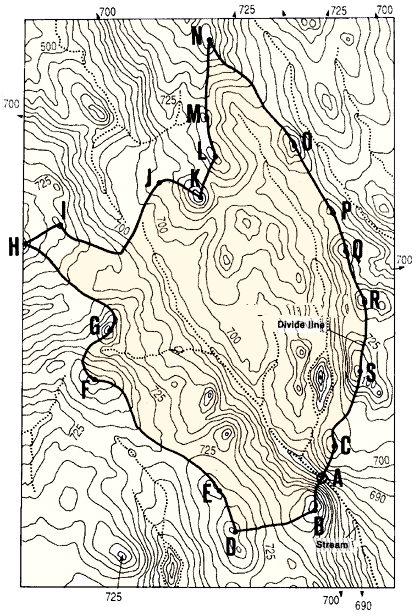6. Using the square-grid method, preferably (see Section 10.3), find the area of the region enclosed by the divide line to find the size of the drainage areafor stream point A.

7. You can estimate the quantity of water available at point A from the size of the drainage area, the most common vegetation there, the general relief and the amount of rainfall in the area. To do this, you need to obtain the values of the local runoff coefficients from a government office, such as the hydrological service.

Example

Water availability per square kilometre of drainage area in the Bouaké region, Cote d'Ivoire, Western Africa

 Annual rainfall (mm) Runoff coefficient (percent) Runoff1 (mm) Water availability2 (m3/km2) 800 0.9 7 7000 900 2.8 25 25000 1000 4.7 47 47000 1100 6.6 72 72000 1150 7.5 87 87000 1200 8.5 101 101000 1300 10.4 135 135000 1400 12.3 172 172000 1500 14.2 212 212000 1600 16.1 257 257000 1700 18.0 305 305000

1 Runoff (mm) = annual rainfall (mm) x (runoff coefficient ÷ 100)
2 Water availability (m3/km2) = runoff (mm) x 1000

As the average annual rainfall in this region amounts to 1 150 mm, you can estimate the average water availabilityto be about 87000 m3/km2 of drainage area for this particular region of the country. If the calculated drainage area upstream from point A is 2.72 km2, the average water availability at point A can be estimated as 87000 m3/km2 x 2.72 km2 = 236640 m3 per year.### Finding the size of the area to be flooded

8. If you need to build a dam at point A to create a water reservoir, you can fairly easily determine the size of the area to be flooded upstream from point A, if you know the elevation of the water surface in the reservoir.

9. At the dam site, identify the contour line on the topographical map which, on one side of the stream, corresponds to the elevation of the reservoir's water surface. Follow this contour line first upstream, then across the stream, and finally downstream, back to the dam site. The area enclosed within this contour line will be the flooded area for that particular reservoir water level.

Example

Elevation of reservoir water surface level: 690 m. Follow the 690 m contour line from point B at the dam site, on the left bank, up to point C (stream crossing) and back down to point D at the dam site, on the right bank. The flooded area at the 690 m water level will be BCDB.10. Using the square-grid method, preferably (see Section 10.3), find the area of the zone within the selected contour line to obtain the size of the flooded area. If you know the average depth of the reservoir, you can then calculate the volume of water which is stored in it.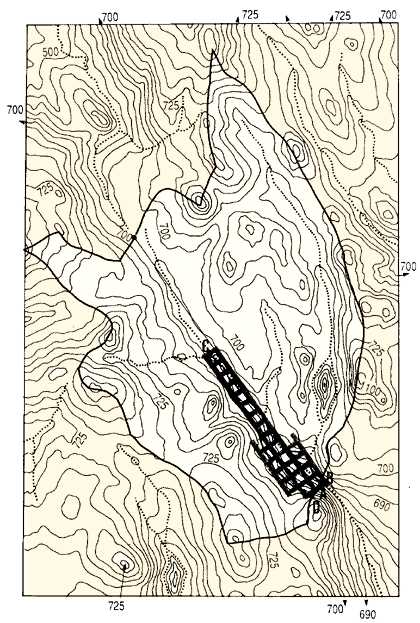### Obtaining ground profiles from topographical maps

11. From a topographical map, you can find the profile of the ground along any selected straight line AB. Draw line AB on the map. Place one edge of a straight strip of white paper along this line, and mark on it the position of the main contour lines 775 m, 750 m ... 675 m which line AB intersects. Next to these marks, note their elevations.

12. Transfer these marks onto square-ruled millimetric paper, using a horizontal distance scaleidentical to the map scale.

13. Make a vertical scale for elevations 10 to 30 times larger than the horizontal scale, and mark this scale according to the contour lines present along the profile.

14. Indicate the elevation of each distance mark by a point along a perpendicular line.

15. Join these points to obtain the ground profile along line AB.

 Profile along line AB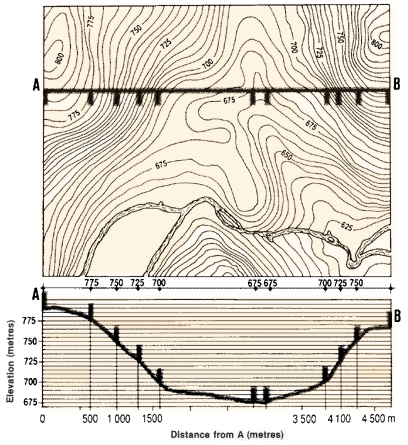### Finding differences in elevation along a stream

16. You may need to choose a site on a stream to build a dike which will create a water storage reservoir, or you may want to use an existing stream to supply a fish-farm with water. In these cases, you should study the longitudinal profile of the stream and determine its slope between two selected points. If you are choosing a dam site, you should study the slope from the dam site to the highest elevation (upper reach) of the future reservoir. This study will give you an idea of the volume of water that can be stored in the reservoir. If you want to divert a stream to supply water, you will measure the slope from the water-intake point on the fish-farm to its water outlet. This study will give you the difference in elevation available for you to build a fish-farm on the site which lies between these two points.Pond site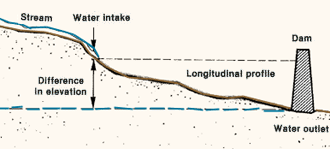17. To determine the slope of the stream between A and B, for example, first clearly mark on the map the two extreme points A and B of the profile you want to study. Then mark points C, D, E and F, at which contour lines cross the stream bed. For later reference, you may also mark points of particular interest along the stream, such as a tributary or branch of the stream (G, H), or a road bridge.

18. Starting at point A, measure to the millimetre, the distancesAG, GC, CD ... FB between these various marked points, closely following the stream bed as you do so. Enter these measurements in a table as shown in the example.

19. Using the map scale, transform these map measurements into ground distances (in metres), and calculate the cumulative distances from point A, as shown in the table in the example.

20. From the contour lines of the map, determine the elevations of points A, G, C, D, H, E, F and B, and enter these figures in the table. As you are working downstream, these altitudes should steadily decrease by a constant value equal to the contour interval of the map.Example

Longitudinal profile of stream section AB

 Stream point Map distance (cm) Ground1 distance (m) Cumulative distance (m) Elevation2 (m) A G C D H E F B 2.9 0.7 1.1 0.4 2.1 0.5 1.1 580 140 220 80 420 100 220 0 580 720 940 1020 1440 1540 1760 690 (tributary) 685 680 (tributary) 675 670 665

1 Ground distance (m) = map distance (cm) x map scale (m/cm).
2 From contour lines with contour interval = 5 m.

Longitudinal profile AB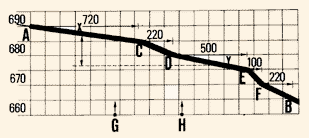21. Draw the longitudinal profile of the AB stream section as explained earlier (see Section 9.5), using the information you have gathered. To its horizontal scale, add the positions of the additional points of interest so that you can refer to them later.

22. From this longitudinal profile, you can now easily determine the difference in elevation existing between any two points X and Y of the stream within this section AB.

### Determining the shape of stream valleys

23. Using a method similar to the one described in steps 11-15, you can also determine the general shape of a stream valley. To do this, you will draw cross-section profiles perpendicular to the stream bed, at points of interest to you. These points will depend on the purpose of your survey. If you are planning to build a small dam, you will draw cross-section AB. If you are looking for a suitable fish-farm site, you will draw cross- section CD.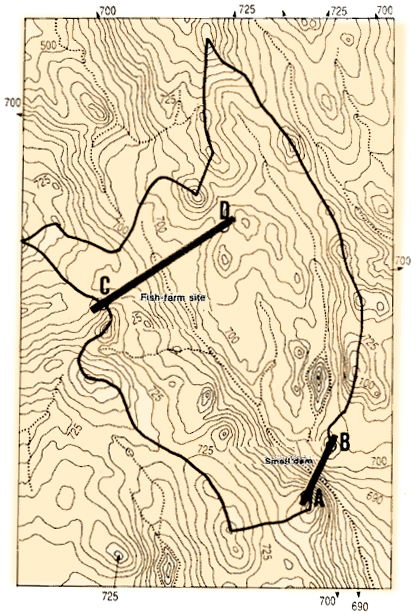24. Get a strip of paper and align one of its edges with the cross-section line. Mark on the strip the positions of the various contour lines, together with a few elevations for reference.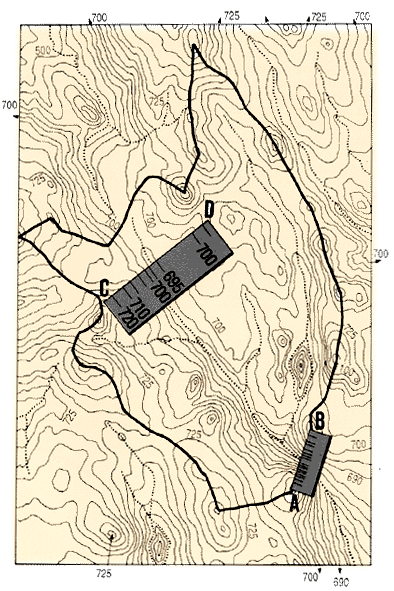25. Transfer these marks onto the horizontal scale of a cross-section profile (see Section 9.5). This scale will be the same as the map scale.

26. Select a vertical scale for the elevations 10 to 30 times larger than the horizontal scale. Transfer the elevations of each mark vertically onto the graph. The line joining the points represents the cross-section profile of the valley along line AB.

27. If you repeat this procedure for line CD, you will draw the valley cross-section profile CD. When comparing it to cross-section AB, you can see that the two profiles are different. Profile AB has a true V-shape, but profile CD has a V-shape deformed on one side.

 Cross section ABCross section CD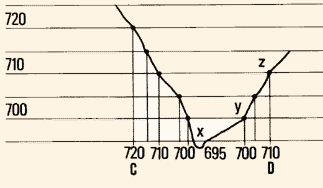28. In the context of fish culture, cross-section valley profiles may be classified into four types, according to their shape. When you know the shape of the valley in the area where you want to build your fish-farm, you will be able to:

• decide which type of pond to build;

This information will be explained to you in the next volume in this series. You will learn, for example, that site AB above would be a good place to build a dam at minimum cost, but it would not be suitable for fish-ponds. At site CD, however, the XYZ side of the valley shows a lateral slope (1.25 to 2.17 percent) suitable for the construction of fish-ponds (see step 27, above).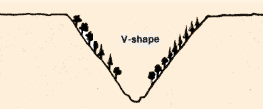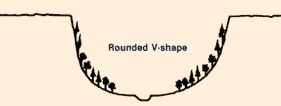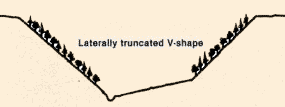### Making a slope map from a topographical map

29. Ground slope is one of the most important elements in the selection of a suitable site for fish-pond construction. The best slope conditions for a fish-pond are on land with a slope averaging 0.5 to 1.5 percent, but conditions on near-to-horizontal ground and on ground with slopes from 1.5 to 3 percent are still fairly good. As the slope increases, the cost of construction increases also, particularly above 5 percent slope.

30. When you study a topographical map, you may find it useful to determine a series of slope categorieson it. In this way, you will have made a slope map.

Example

Ground slope categories useful in fish culture

A - ground slope smaller than or equal to 1.5 percent
B- ground slope between 1.5 and 3 percent
C - ground slope between 3 and 5 percent
D - ground slope greater than 5 percent

31. To prepare a slope map, you must first make contour line-spacing guides for each of the slope categories and for the particular topographical map you are using. If this topographical map has:

• a distance reduction scale where 1 cm = n (m)
• a contour interval Cl(m)

the interval X (in cm) between the lines of your spacing guide is calculated as:

 X = (100 Cl) ÷ (nS)

S being the greatest slope in percent characterizing each of the slope categories you want to map.

 Usefulness of slopes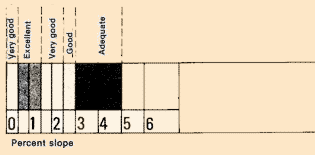Categories for slope map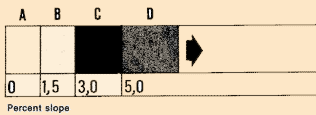Example You have a topographical map on which you want to map the above four slope categories (A, B, C, D). You first need to make three spacing guides for 1.5, 3 and 5 percent slopes, respectively. The topographical map scale is 1 :50000 (1 cm = 500 m) and the contour interval is 5 m. Calculate the intervals X between the lines of your spacing guides as follows: 1.5 percent slope: X = (100 x 5) ÷ (500 x 1.5) = 500 ÷ 750 = 0.67 cm or 6.7 mm 3 percent slope: X = (100 x 5) ÷ (500 x 3) = 500 ÷ 1500 = 0.33 cm or 3.3 mm 5 percent slope: X = (100 x 5) ÷ (500 x 5) = 500 ÷ 2 500 = 0.2 cm or 2 mm 32. Get several sheets of squared-ruled millimetric paper and prepare your spacing guides as follows: using a sharp pencil with a hard lead, draw a line across the sheet of paper close to the bottom; for a vertical distance of about 15 cm, draw a series of lines parallel to this bottom line, at a distance equal to the interval X (calculated as explained above), for example, 6.7 mm for the 1.5 percent slope. 1.5 percent is your spacing guide; up to a further vertical distance of about 10 cm, draw a second series of lines parallel to the first series, at a distance equal to the interval X calculated for the second slope value, for example, 3.3 mm for the 3 percent slope. 3 percent is your spacing guide; repeat the procedure to obtain the 5 percent spacing guide.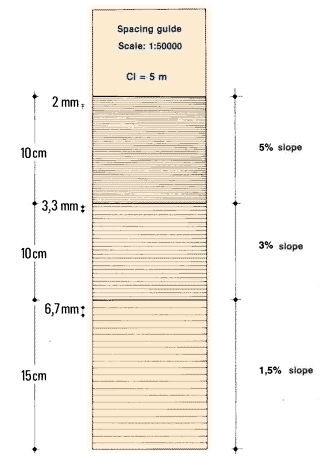33. You are now ready to make your slope map, identifying, for example, the four slope categories on the topographical map. Proceed in the following way: (a) Get several differently coloured crayons or coloured pencils. Select one colour to represent each slope category. The lightest colour can represent the least sloping ground (0 to 1.5 percent), while the darkest colour can be for the most sloping ground (more than 5 percent). (b) Cut a 2 cm strip off your contour-line spacing guide (see step 32) which gives the minimum interval between contour lines on your topographical map for the "1.5 to 3 percent" category, the "3 to 5 percent" category, and the "greater than 5 percent" category. Cut the strip perpendicular to the spacing lines so that all three categories are shown on it.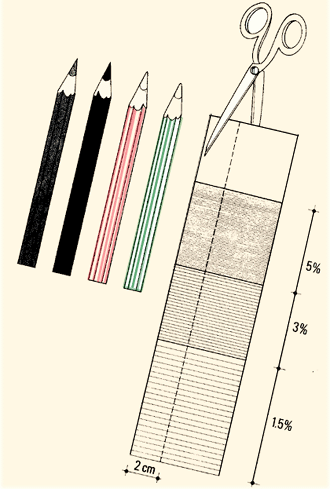(c) Place this strip on the map. Then, going through them one by one, determine which sections have intervals between the contour lines smaller than or equal to the interval on the strip section that corresponds to the 5 percent spacing guide. Since such sections have slopes equal to or greater than 5 percent, you should colour them with the darkest colour.

Note: you should compare the interval of the strip with the intervals between contour lines along a line perpendicular to the contour lines, which is the direction of maximum slope.

(d) Repeat the same procedure with the section of the strip corresponding to the 3 percent spacing guide. Determine which uncoloured sections of the map have intervals between contour lines smaller than or equal to the strip interval. Such sections have slopes from 3 to 5 percent, and you should colour them with the next lightest colour.

(e) Repeat this procedure with the section of the strip that corresponds to the 1.5 percent spacing guide, and determine the uncoloured sections of the map which have slopes from 1.5 to 3 percent. Colour them with the next lightest colour.

(f) Finally, using the same procedure, check that the uncoloured sections of the map have intervals between the contour lines greater than the interval on the 1.5 percent section of the strip. Colour these sections with slopes less than 1.5 percent with the lightest colour of all.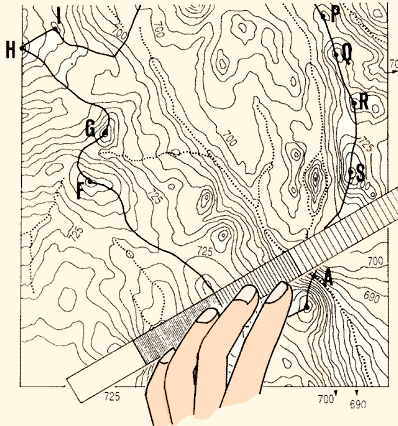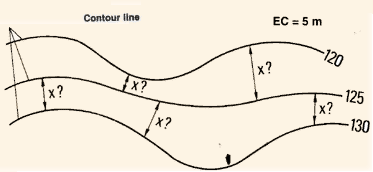## 11.2 How to make a reconnaissance survey of a possible site

1. After you have made preliminary studies from available topographical maps, you can choose the best potential site(s) for the construction of fish-ponds (see the next volume in this series).

2. You should now organize a reconnaissance survey of the selected site(s) in the field in order to obtain more detailed topographical information. This survey should include, at least, a longitudinal profile of the stream valley and/or the selected site(s), as well as cross-section profiles of the site(s). If you are planning the construction of a reservoir, you can survey its maximum area by contouring and find its maximum water level by levelling. Then, you can calculate both the surface area and the water volume of the reservoir.

Longitudinal profileCross-section profile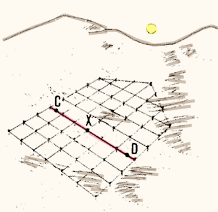Contouring### Studying the longitudinal profile of the stream valley

3. First, make a levelling survey to obtain the longitudinal profileof the stream valley (see Section 8.2). To do this, traverse along a series of straight lines closely following the stream. Plot the profile on graph paper (see Section 9.5). Then you can calculate the difference in elevationbetween any two points of the longitudinal profile, such as the future water intake F and the future water outlet A. This difference in elevation E(F)-E(A) should be great enough to allow you to build the proposed fish-farm (see next volume in this series).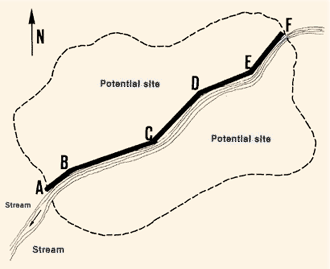4. If you are planning to build a reservoir, you can use a similar procedure to easily estimate the approximate height of the dam at point A, together with the maximum water depth of the future reservoir.

Reservoir AF### Making cross-section profiles of the potential site

5. You survey a series of cross-section profiles GH, IJ, ...,based on the above longitudinal profile, across a valley at intervals of 20 to 50 m, covering the entire area of the potential site (see Section 8.2). Plot the profiles on graph paper. This makes it possible to calculate the ground slopes and, on this basis, design the fish-farm.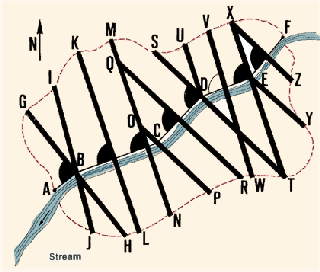### Contour-mapping of the potential site

6. From the results of the above survey, you can also prepare a plan of the potential site, and plot the contours on it (see Section 9.4, step 10).

7. In another contour mapping method, you first choose a reference pointon the potential site, such as the water intake point A at the stream. Point A will then be used as a bench-mark with a given elevation E(A) = 100 m, for example: from point A, you will identify the E(A) contour ABC ... H in the potential site, by contouring (see Section 8.3).

8. At intervals of 20 to 50 m on this E(A) contour, you will survey perpendicular cross-section profiles from the contour to the stream. From the results of this survey you can identify other contours and prepare a contour map.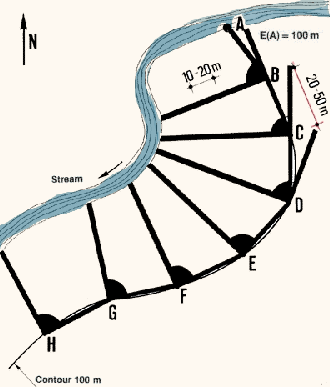### Finding the maximum area of a reservoir

9. When you have fixed the positions of both the water inlet A and the dam centre-line XY of a proposed reservoir, you can easily determine, on the ground, the maximum area of this future reservoir. From the water inlet A, find the position of contour E(A) by contouring from A on both stream banks to line XY.## 11.3 How to make a feasibility study of a potential site

1. From the data obtained during the reconnaissance surveys, you can draw a topographical plan to scale, showing the various distances and elevations. You can study the design of the fish-farm or, if you need to build a dam, you can obtain additional information on the characteristics of the water reservoir that will result from the dam. Fish-farm design will be discussed further in the next volume in this series, and you will learn more about the characteristics of reservoirs there. In this section you will learn how to estimate the volume of a dike built from earth, and how to calculate height differences for pumping stations.

### Estimating the area of the future reservoir

2. On your topographical plan, transfer the position of the E(A) contour which encloses the maximum area of the future reservoir (see step 9 above). You may then estimate the size of the area enclosed by the E(A) contour line, using measurements on the map.### Estimating the volume of the future reservoir

3. In Water for Freshwater Fish Culture (Volume 4, Section 4.2), you learned simple methods for determining the volume of a future reservoir. Now, you will learn a fairly accurate, rapid field method based on topographical surveys.

4. Every 20 to 50 m along the longitudinal axis AZ of the future reservoir, mark perpendicular lines BC, DE ... QR within the area enclosed by contour E(A). Use wooden pegs at 25 m intervals along the perpendiculars, and mark them on each side of the axis AZ.

5. Start from end-point B of line BC, on contour E(A) where the maximum water level will line up with the ground elevation. Using a target levelling staff and a sighting level, transfer elevation E(A) to the top of the pegs a, b and c. Drive them into the ground until they are at the correct elevation. Pegs a, b and c now clearly show the maximum depth of water which will be present at each of these points when the reservoir is full.

6. Repeat this procedure for each transversal line DE, FG ... QR successively; similarly, stake out XY, the centre line of the proposed dike.7. In a simple table, measure and record the height of each peg above ground level for each transversal line, including line XY, as shown in the example.

Example

 Line Peg height, m a b c d e BC 0.45 0.87 0.38 - - DE 0.85 1.42 0.73 - - FG 0.22 0.87 1.63 0.79 - KL 0.49 0.98 1.89 0.91 0.58 ... ... ... ... ... ... XY 0.82 2.42 0.84 - -

8. Using the correct scale, draw the cross-sections BC, DE ... QR, and XY of the completely filled reservoiron square-ruled millimetric paper. Use a vertical scale 10 times larger, for example, than the horizontal distance scale. Remember that the end-points of each cross-section have, by definition, a zero depth of water.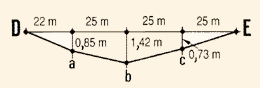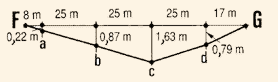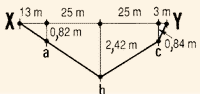9. Calculate the area of each cross-section, adding the partial areas of triangles and trapeziums as necessary (see mathematical formulas in Annex 1).

 Area BCExample

Area BC = triangle 1 + trapezium 2 + trapezium 3 + triangle 4

 Triangle 1 = (17 m x 0.45 m) ÷ 2 =  3.825 m2 Trapezium 2 = [(0.45 m + 0.87 m) ÷ 2] x 25 m = 16.500 m2 Trapezium 3 = [(0.87 m + 0.38 m) ÷ 2] x 25 m = 15.625 m2 Triangle 4 = (13 m x 0.38 m) ÷ 2 =   2.470 m2 Area BC = 38.420 m2

10. Add the areas of cross-sections BC, DE ... QR, and multiply this sum by the fixed interval between cross- sections (in this case, 25 m) to obtain an estimate of the volume of the reservoir upstream from the last cross- section QR.

Example

Volume of reservoir from point A to line QR = (area BC + area DE + ... + area QR) x 25 m

11. Estimate the volume of the last section of the reservoir, between cross-section QR and the dike's centre-line XY. Multiply the area of cross-section XY (see step 9) by half the distance between previous cross-sections.

Example

Volume section QR/Z = (area XY) x (25 m ÷ 2)

12. Calculate the volume of the entire reservoir by adding:

• the volume A/QR obtained in step 10; and
• the volume of QR/Z obtained in step 11.

Example

Volume A/Z = volume A/QR + volume QR/Z

### Estimating the volume of an earth dam

13. To rapidly estimate the volume of an earth dike XYto be built across a given valley, use the following method. It will provide a volume estimate about 10 percent smaller than the actual volume. But this level of accuracy is good enough for the initial estimate.

14. Using the information from the table in step 7, calculate the heights h of the dam XY at mid-points between consecutive pegs.

Example

For the dam XY there are only two mid-points to consider: one between pegs a and b, and one between pegs b and c.

• Dike height a/b = (0.82 m + 2.42 m) ÷ 2 = 1.62 m
• Dike height b/c = (2.42 m + 0.84 m) ÷ 2 = 1.63 m

Mid-points to calculate the volume of dam XY15. Using the correct scale, draw the transversal section of the type of dike you plan to build (see Constructions, 20 in this series). There are three measurements, in particular, which you must determine:

• the width C of the highest point, or crown, of the dike;
• the dry-side slope of the wall outside the reservoir, D: 1;
• the wet-side slope of the wall inside the reservoir, W: 1.

16. If you know these characteristics of the dike, you can calculate the area of any transversal section of this dike by adding:

• rectangle 1 area = C x h;
• triangle 2 area = (D x h) x (h ÷ 2);
• triangle 3 area = (W x h) x (h ÷ 2).

Therefore, the area A of any transversal section of the dike equals:

 A = (Ch) + (Dh2 ÷ 2) + (Wh2 ÷ 2)

where C is the crown width of the dike, h the height, Dthe dry slope, and W the wet slope.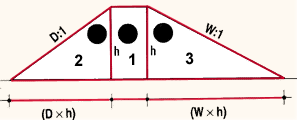17. Apply the above formula to successively calculate the section area of the dike at each of the XY mid-points, using the h-values obtained in step 14.

Example

It the dike characteristics are fixed as follows: C = 4 m; dry slope = 1.5: 1; wet slope = 2: 1;

the dike's transversal section areas are:

• At mid-point a/b where h1 = 1.62 m
A1= (4 m x 1.62 m) + (1.5 x 1.622 m) ÷ 2 + (2 x 1.622 m) ÷ 2 = 6.48 m2 + 1.97 m2 + 2.62 m2 = 11.07 m2
• At mid-point b/c where h2 = 1.63 m
A2 = (4 m x 1.63 m) + (1.5 x 1.632 m) ÷ 2 + (2 x 1.632 m) ÷ 2 = 6.52 m2 + 1.99 m2 + 2.66 m2 = 11.17 m2

18. Calculate the partial volumes of each portion of the dike marked by pegs a, b, c, etc. To do this, multiply each corresponding mid-point section area by the length of the dam portion.

Example

Partial volumes of the dike with pegs a, b and c at 25 m intervals:

• For portion a/b V1 = A1 x 25 m = 11.07 m2 x 25 m = 276.75 m3
• For portion b/c V2 = A2 x 25 m = 11. 17 m2 x 25 m = 279.25 m3

19. Obtain the estimate of the total volume of the dikeby adding these partial volumes.

Example

Total volume of dike XY = 276.75 m3 + 279.25 m3 = 556 m3

### Using what you know about topography to install a pumping station

20. You may be planning to pump water for your reservoir either from a well or from an existing body of water. If so, the kind of pump you choose will greatly depend on the difference in elevation between the two extremities of your water pipeline and the pump. Usually, you will site the pump at an intermediate elevation, where it can bring the water up by suction from a lower elevation (the source of water) and force it on to a higher elevation (a reservoir tank, for example).

21. When you are choosing a site for your pumping station, two differences in elevation are particularly important:

• From the water-source surface to the pump, the suction head(in metres);
• From the pump to the reservoir, the delivery head (in metres).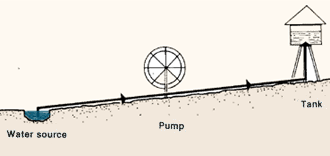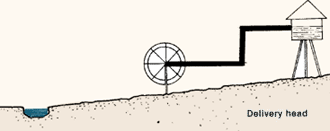22. To obtain these differences in elevation, you may use direct levelling from the water source A to the pumping station B, and to the site C of the reservoir tower. Then, knowing the ground elevations at these points and the various vertical distances (for example, water source/pump axis and pump axis/tank water), you can easily calculate the suction head and the delivery head.

23. You can instead use an indirect method to find the differences in elevation:

• measure the vertical angles made with the horizontal plane by successively sighting lines AB and BD (see Chapter 4);
• measure horizontal distances AE and BC (see Chapter 2);
• calculate the differences in elevation, as follows:
• EB = AE tan BAE
• CD = BC tan DBC,
• obtaining the tangent values from Annex II.
 Direct levelling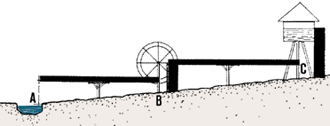Measure angles BAE and DBC...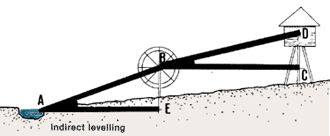...then measure horizontal distances AE and BC and calculate the differences in elevation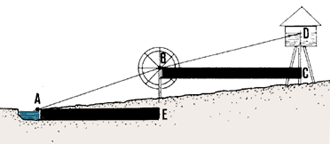## 11.4 How to make levelling surveys for fish-farm construction

1. You have already learned that you need to know how to use the right topographical methods during the construction of your fish-farm. Now you will learn about two additional topographical methods, one for the construction of a water-supply canal and one for the construction of a pond.

### Staking out a water supply canal for construction

2. You learned, in earlier chapters, how to first survey the centre-line of a water-supply canal (see Sections 7.1 and 8.2), then how to draw its longitudinal profile (see Section 9.5) and its cross-section profile (see Section 9.6). You have also learned how you can contour (see Section 8.3) to rapidly identify the route a canal can take between the water intake point and the fish-farm water inlets.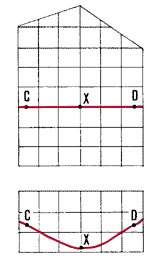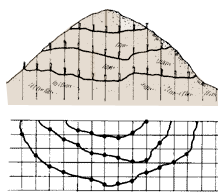3. Once the route of the water-supply canal has been carefully defined and identified in the field, you need to stake it out before you can begin building it. To do this, you will first have to clear a 1 to 2 m stretch of land along the canal centre-line. Then you will set out a series of short pegs along this centre-line. The summits of the pegs must correspond to a horizontal reference level, that is, the tops must all be at the same height.

4. The distance between these reference pegs depends on the levelling method you are using. Usually, the simplest method to use is to proceed from the starting point A with a 4 m straight edge and a mason's level (see Section 6.6). But you could also use a clisimeter (see Section 4.5) and a target levelling staff. In this case, the pegs are placed at 5 to 10 m intervals.

5. Then, define the cross-section profile of the canal. (see Constructions, 20, in this series) Add pegs as you need them, to direct the workers in their digging.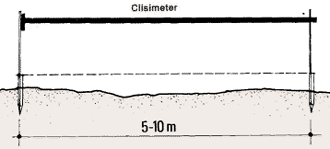Cross section6. If you are building a canal without a bottom slope, you can show the workers the fixed distance to which they must dig by giving them a wooden stake the same length as the top level of the centre-line pegs.

7. If you are building a canal with a bottom slope, the simplest method you can use is to give a similar slope to the horizontal reference level which joins the top of the centre-line pegs. To do this, proceed in the following way:

(a) From the value of the slope and the distance between consecutive stakes, calculate how much difference in elevation should exist from one peg to the next one.

Example

Using a straight-edge and mason's level for levelling, place your pegs at 4 m intervals. If the slope of the canal bottom is to be, for example, 0.1 percent, the difference in elevation between one peg and the next equals (0.1 m x 4 m) ÷ 100 m = 0.004 m = 4 mm.

 Canal bottom, no slopeSetting slope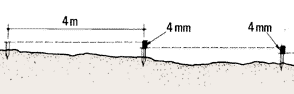(b) Cut a small piece of wood of the same thickness as this difference in elevation.

(c) Put this piece of wood on top of the second peg, and drive this peg deeper into the ground until the top of the first peg and the top of this second one are again horizontal. Use a straight-edge and mason's level, for example, to guide you in doing this.

(d) Move to the third peg, put the piece of wood on top of it, and drive the peg into the ground until the wood is horizontal again, between the top of the second peg and the top of this one.

(e) Repeat this procedure until you reach the end of the canal centre-line.

(f) The line joining the top of the centre-line pegs now has a slope equal to the required slope of the canal bottom. You can dig the canal as in step 6, using a constant reference length from the top of each peg.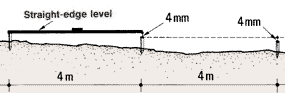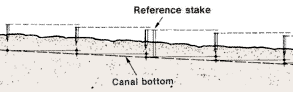### Staking out a pond bottom before construction

8. You have already learned how to survey the periphery, or boundary line, of your ponds by setting out a series of rectangular areas and the centre-lines of the dikes you need to build (see Section 3.7) or by contouring at the maximum water level (see Section 11.2).

9. When you have found the area of your ponds, but before you start building them, you will usually need to know how much earth you will have to remove at the various points of the pond. You will also need to indicate this clearly on the site so that the workers can proceed with the pond construction correctly. To do this, you will need to stake out the bottom of each pond.10. One simple way of staking out a pond bottom is to make a survey by radiation from the lowest point of the future pond, its water outlet. Proceed in the following way.

(a) From point 0 (the lowest point of the future pond), mark a series of lines radiating over the major part of the pond bottom area, using pegs.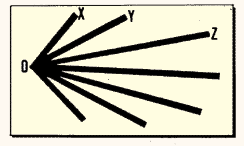(b) Starting with line OX, for example, place a series of pegs along it at fixed intervals. The size of these intervals will vary according to the levelling method you use. For example, it will be 4 m for the straight- edge/mason's level method, and 5 to 10 m when you use a sighting level.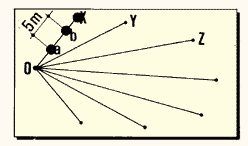(c) Starting from point 0, survey line OX and put the top of all of the pegsat a horizontal reference level.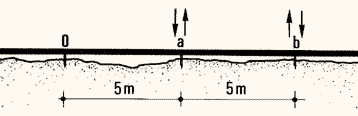(d) Repeat the same procedure for all the marked radiating lines; in this way, you will obtain a series of pegs whose tops are all at the same reference level, and which are dispersed all over the pond bottom area.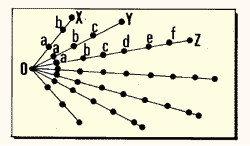(e) On each peg you must now clearly indicate the vertical distance from the top of the peg to the bottom of the pond.(f) From the slope which the pond bottom will have (see Constructions, 20, in this series) and from the distance between consecutive pegs on each radiating line, calculate the difference in elevation necessary from one peg to the next one in each line.

Calculate the difference in elevation for the desired slopeExample

• Slope of pond bottom is to be 1 percent.
• Distance between stakes is 5 m.
• Difference in elevation should be (1 m x 5 m) ÷ 100 m = 0.05 m = 5 cm.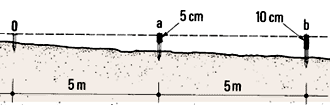(g) From the fixed elevation to be given to point 0 at the pond outlet (see the next volume in this series), and from the surveyed elevation of the top of the peg set out at that point, calculate the difference in elevation required at 0 from the top of the peg. Mark this difference clearly on stake 0.

Example

• Elevation to be given according to construction plans to point 0 = 102.53 m.
• Actual elevation of the top of peg 0 obtained by levelling from bench-mark = 103.43 m.
• Difference in elevation required at 0 = 103.43 m - 102.53 m = 0.90 m = 90 cm.(h) Starting with line OX at point 0, calculate the difference in elevation between the peg top and the pond bottom for each successive peg. Subtract the difference obtained above in (f) each time. Repeat the same procedure for all the other radiating lines. To make your measurements clearer, you can use a simple table as shown in the example. Clearly mark these values on the pegs. When the workers dig the pond, they will use the values as a reference guide.
Line OZExample

Difference in elevation between peg tops and pond bottom, in centimetres

 Line Peg 01 a b c d e f OX 90 85 80 - - - - OY 90 85 80 75 - - - OZ 90 85 80 75 70 65 60 ... ... ... ... ... ... ... ...

1At point 0, the difference in elevation is 90 cm from plans. The fixed difference to be subtracted is 5 cm, see above.ANNEX I

Some useful mathematical formulae for regular geometrical figures
PERIMETER LENGTH AND SURFACE AREA

 Figure Perimeter (P) Area (A) Square a P = 4a A = a2 Rectangle ab P = 2a + 2b A = ab Right-angle triangle abc P = a + b + c A = ab ÷ 2 Trapezium abcdh (a parallel to c) P = a + b + c + d A = (a + b) (h ÷ 2) Circle rd P = 6.28r P = 3.14d A = 3.14r2 A = 3.14d2 ÷ 4

SIDE LENGTH OF RIGHT-ANGLE TRIANGLE

 ABC abc c = a cos ABC  b = a sin ABC  c = Ö(a2-b2) b = c tan ABC

Note: tan - see Annex II; cos - see Annex III; sin - see Table 14

 ANNEX II Tangents and values of angles (Tan = angles expressed in degrees d and minutes m)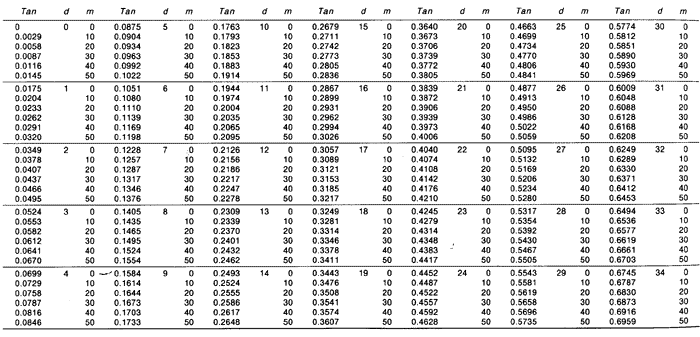ANNEX Ill Cosine values of angles (d = degrees, m = minutes, cos = cosine, x = difference) MAIN TABLE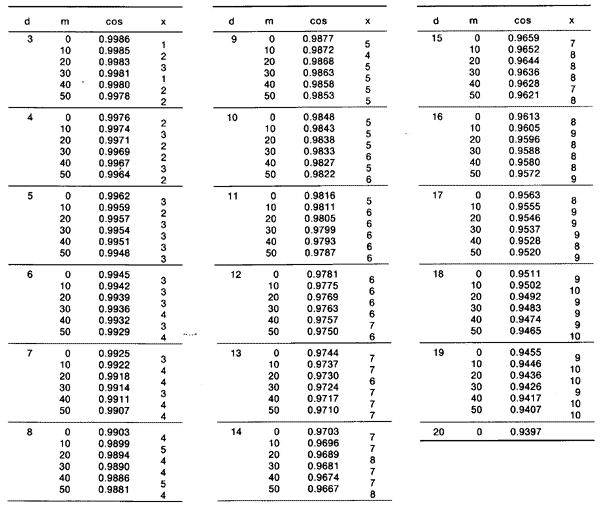TABLE OF PROPORTIONAL PARTS, PExample

To calculate intermediate cosine values using the proportional parts, for cos 7°38' for example, proceed as follows:

• from the Main Table, calculate cos 7°30' = 0.9914;
• obtain the difference between this value and the next, x = 3;
• find column 3 in Table of Proportional Parts, P;
• move down this column to line m = 8, to find P = 2.4;
• subtract P from the last number (4) of the value read from the Main Table, 0.9914 - 0.00024 = 0.99116. This is cos 7°38'.